# 3 Linear Programming 3 1 Linear Algebra Square

• Slides: 423. Linear Programming 3. 1 Linear Algebra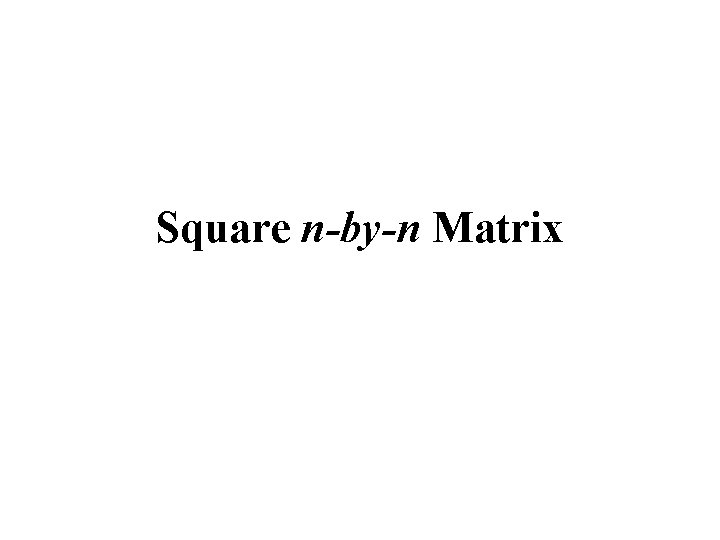Square n-by-n MatrixSolution of Simultaneous Linear Equations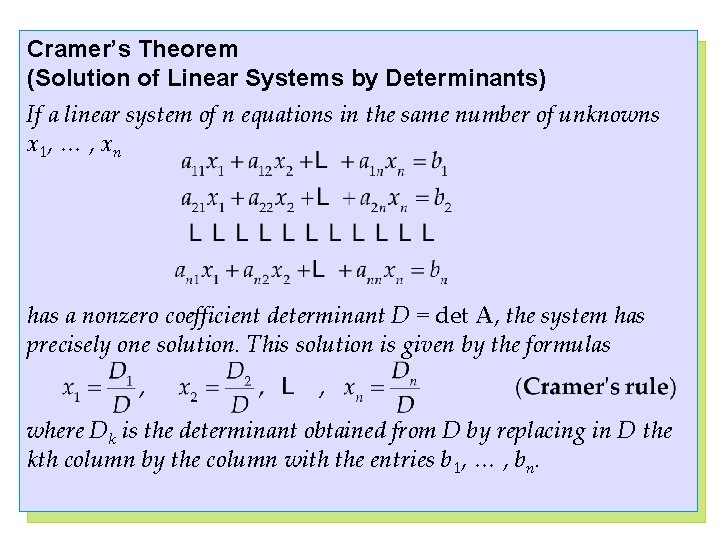Cramer’s Theorem (Solution of Linear Systems by Determinants) If a linear system of n equations in the same number of unknowns x 1, … , xn has a nonzero coefficient determinant D = det A, the system has precisely one solution. This solution is given by the formulas where Dk is the determinant obtained from D by replacing in D the kth column by the column with the entries b 1, … , bn.Gaussian Elimination Forward Elimination pivot 2 u+ v+ w= 1 4 u+ v = -2 -2 u + 2 v + w = 7 Backward Substitution (1) (2) (3) Step 1: equation (2) +(– 2) x equation (1) Step 2: equation (3) + (+1) x equation (1) 2 u+ v+ w = 1 (4) v -2 w =-4 (5) pivot (-1) 3 v+2 w = 8 (6) Step 3: equation (6) + (+3) x equation (5) 2 u+ v+ w = 1 (7) - v - 2 w = -4 (8) - 4 w = -4 (9) w=1 v=2 u = -1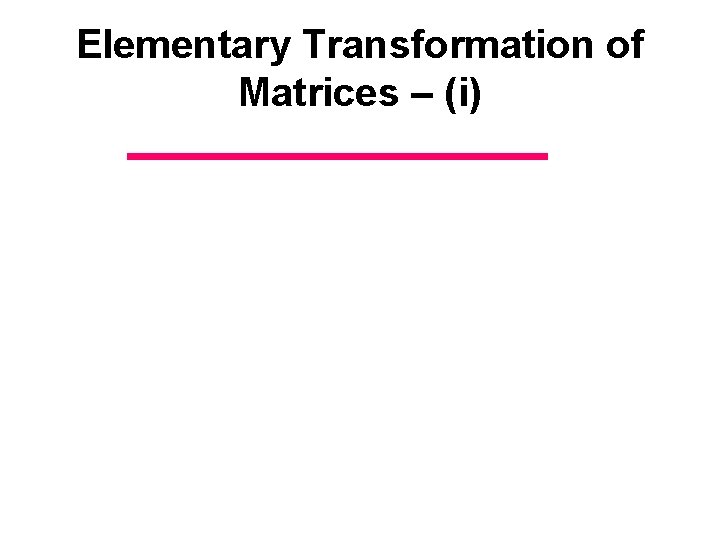Elementary Transformation of Matrices – (i)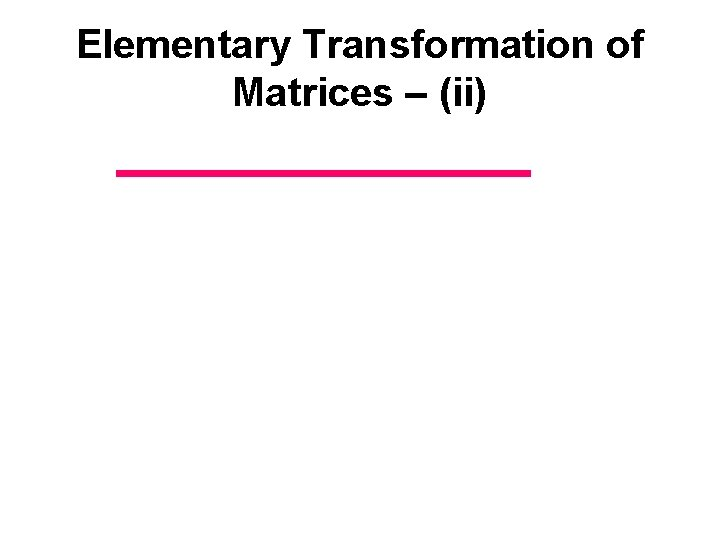Elementary Transformation of Matrices – (ii)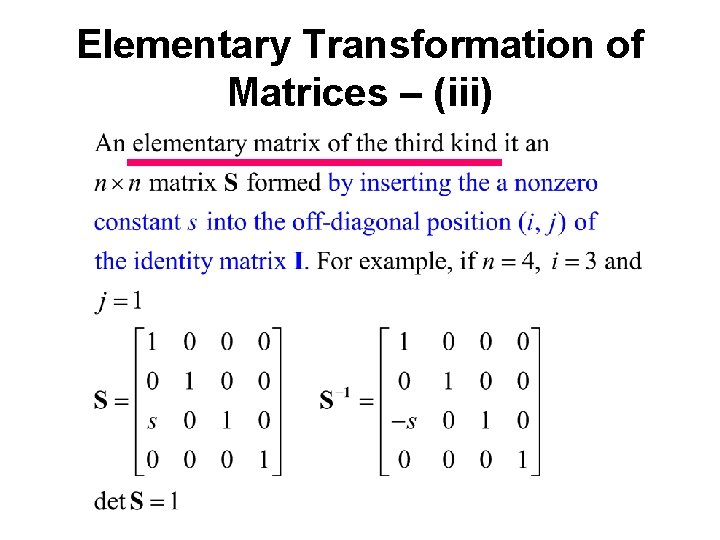Elementary Transformation of Matrices – (iii)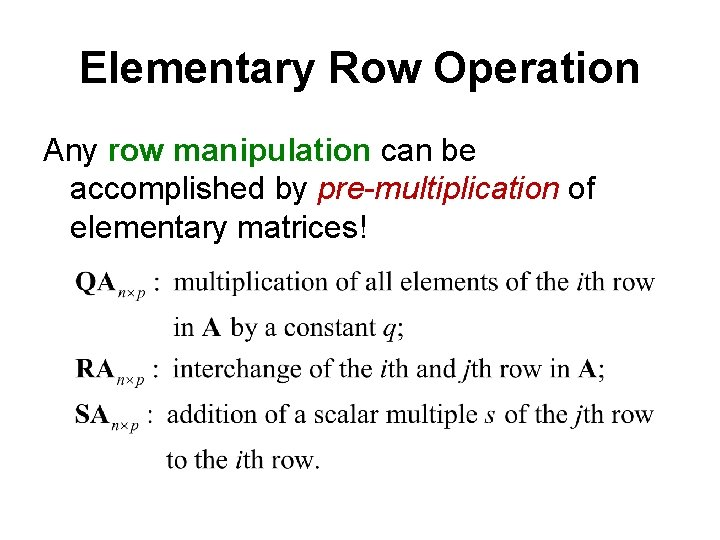Elementary Row Operation Any row manipulation can be accomplished by pre-multiplication of elementary matrices!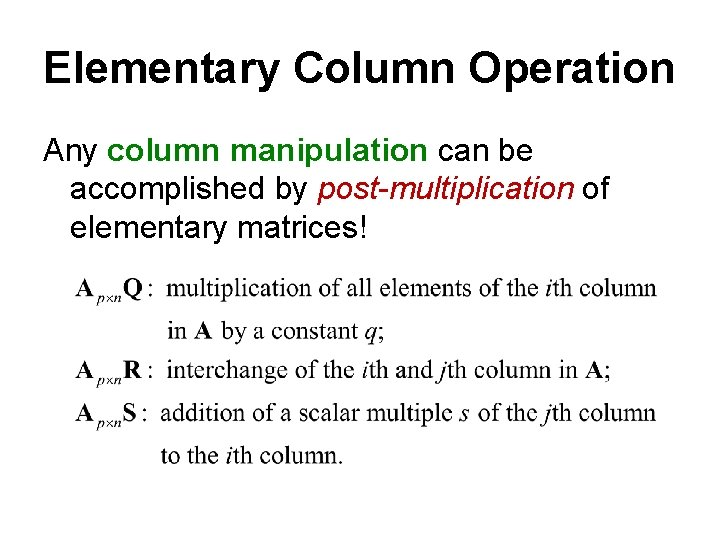Elementary Column Operation Any column manipulation can be accomplished by post-multiplication of elementary matrices!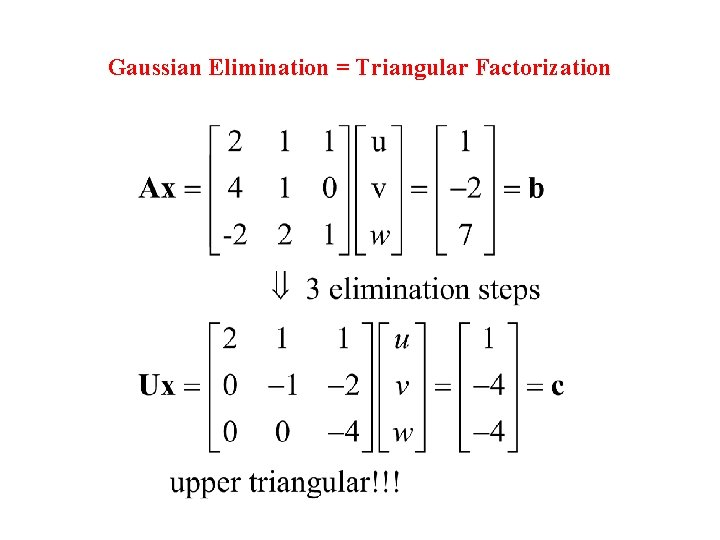Gaussian Elimination = Triangular FactorizationUpper triangular Lower triangularConclusions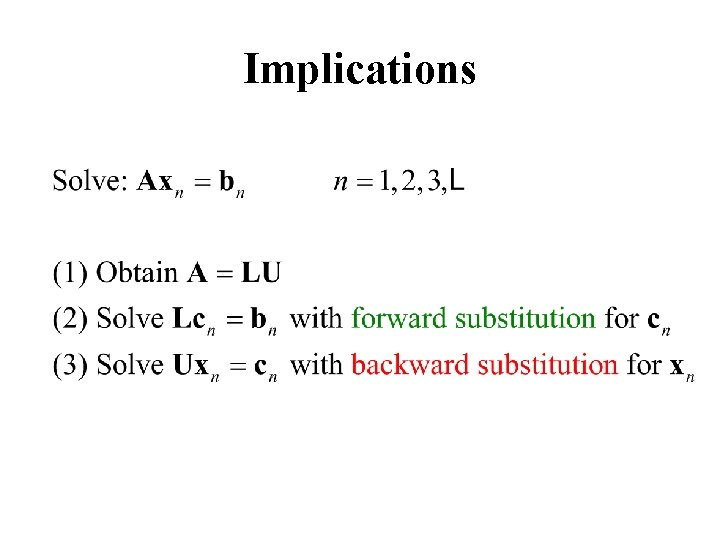ImplicationsRow ExchangeElimination with Row ExchangeRound Off ErrorSingular matrix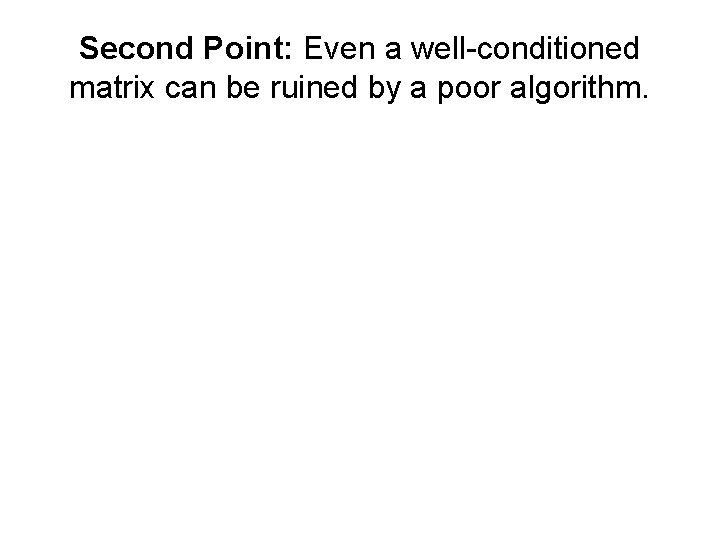Second Point: Even a well-conditioned matrix can be ruined by a poor algorithm.If a calculator is capable of keeping only 3 digits, then Gaussian elimination gives the wrong answer!!! 0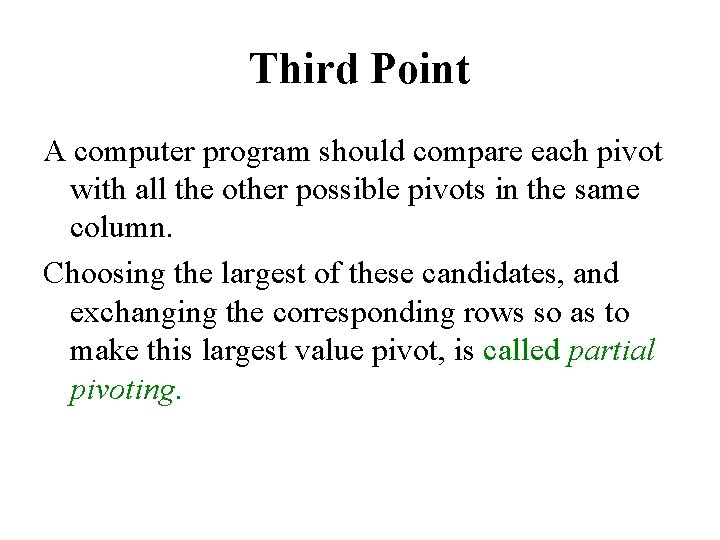Third Point A computer program should compare each pivot with all the other possible pivots in the same column. Choosing the largest of these candidates, and exchanging the corresponding rows so as to make this largest value pivot, is called partial pivoting.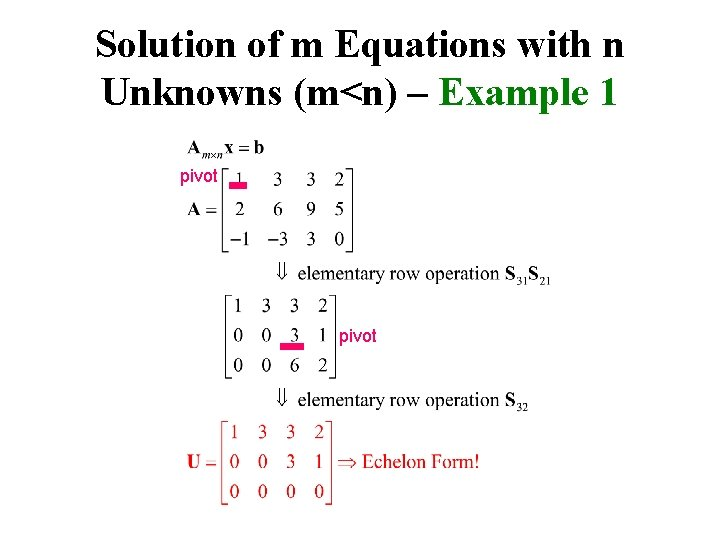Solution of m Equations with n Unknowns (m<n) – Example 1 pivotEchelon FormSolution of m Equations with n Unknowns (m<n) – Example 2 pivot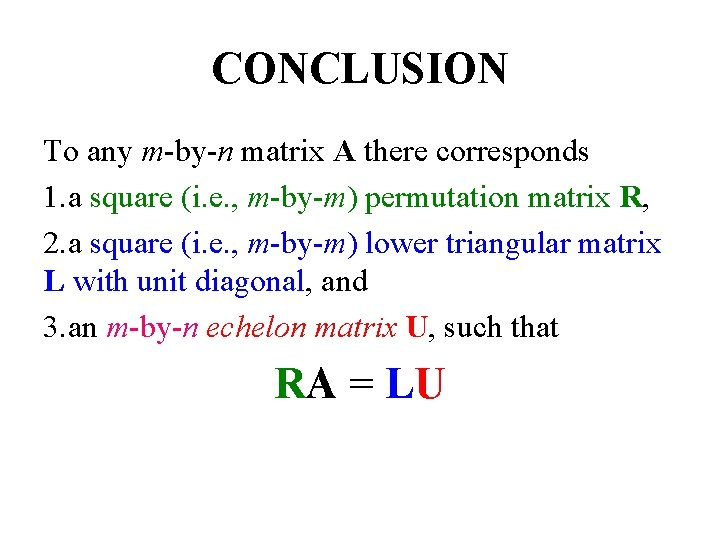CONCLUSION To any m-by-n matrix A there corresponds 1. a square (i. e. , m-by-m) permutation matrix R, 2. a square (i. e. , m-by-m) lower triangular matrix L with unit diagonal, and 3. an m-by-n echelon matrix U, such that RA = LUHomogeneous Solution for Example 1 pivot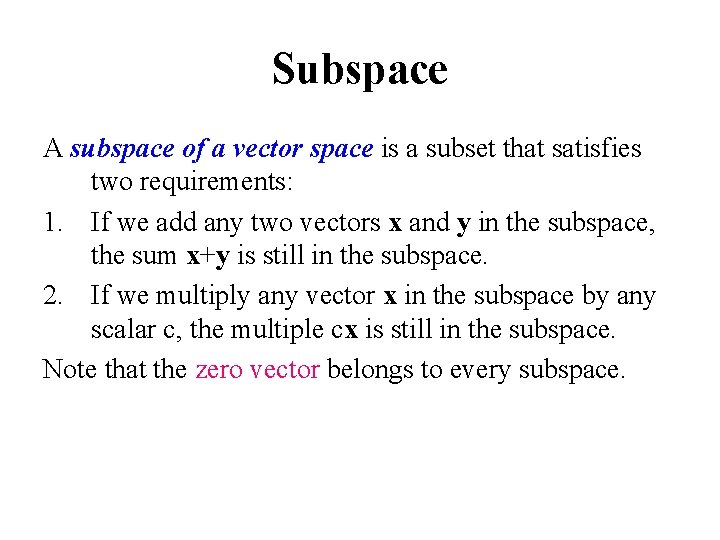Subspace A subspace of a vector space is a subset that satisfies two requirements: 1. If we add any two vectors x and y in the subspace, the sum x+y is still in the subspace. 2. If we multiply any vector x in the subspace by any scalar c, the multiple cx is still in the subspace. Note that the zero vector belongs to every subspace.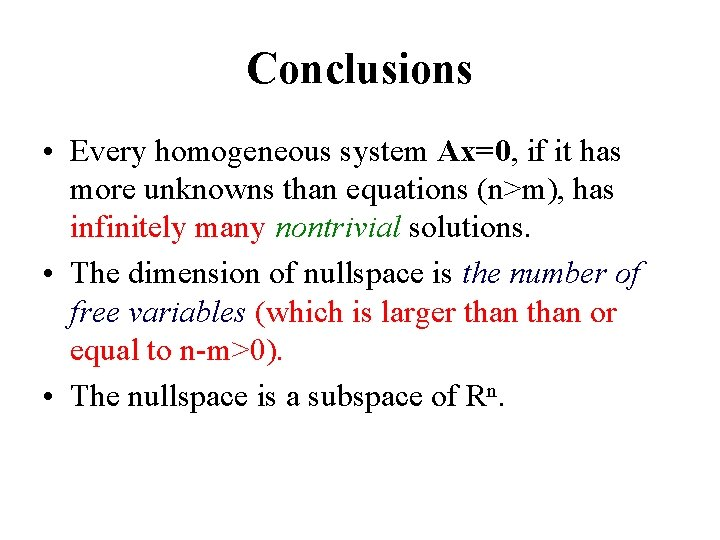Conclusions • Every homogeneous system Ax=0, if it has more unknowns than equations (n>m), has infinitely many nontrivial solutions. • The dimension of nullspace is the number of free variables (which is larger than or equal to n-m>0). • The nullspace is a subspace of Rⁿ.Inhomogeneous Solution of Example 1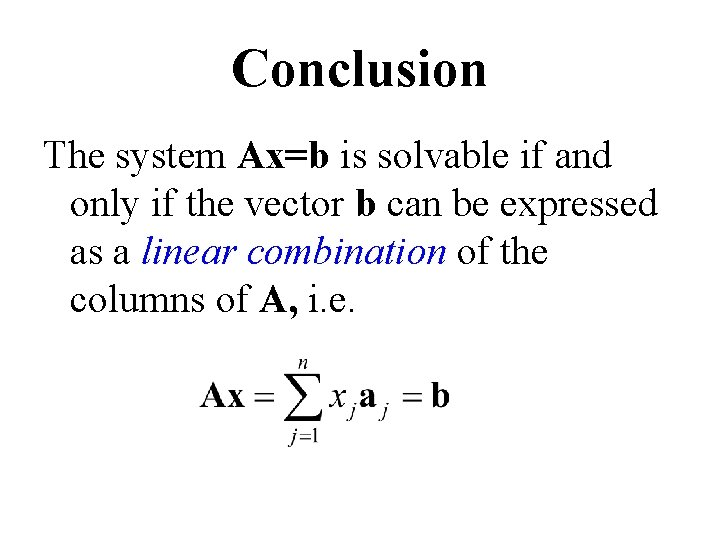Conclusion The system Ax=b is solvable if and only if the vector b can be expressed as a linear combination of the columns of A, i. e.Conclusionshomogeneous solution Ax=0 particular soln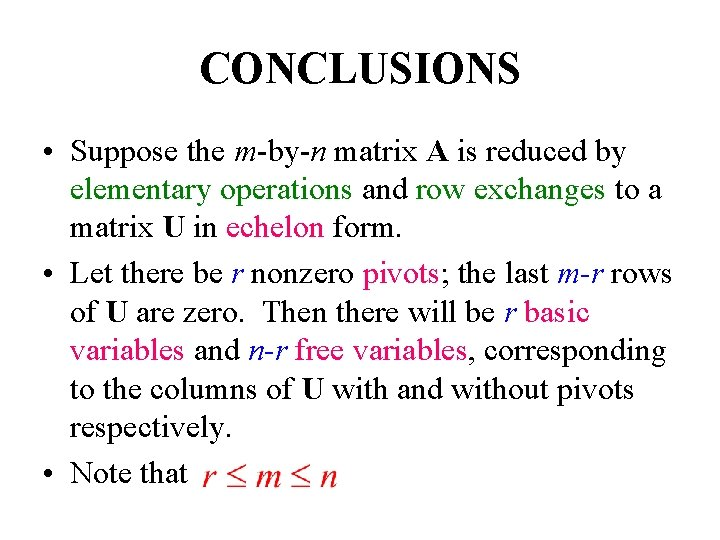CONCLUSIONS • Suppose the m-by-n matrix A is reduced by elementary operations and row exchanges to a matrix U in echelon form. • Let there be r nonzero pivots; the last m-r rows of U are zero. Then there will be r basic variables and n-r free variables, corresponding to the columns of U with and without pivots respectively. • Note thatCONCLUSIONS • The nullspace, formed of solutions to Ax=0, has the n-r free variables as the independent parameters, i. e. , its dimension is also n-r. If r=n ( ), there are no free variables and the null space contains only x=0. • Solution always exists for every right side b iff r=m<n. In this case, since U has no zero rows, Ux=c can be solved by back-substitution.CONCLUSIONS • If r<m<n, then U will have m-r zero rows and there are m-r constraints on b in order for Ax=b to be solvable. If the particular solution exists, then every other solution differs from it by a vector in the nullspace of A. • The number r is called the rank of the matrix A.